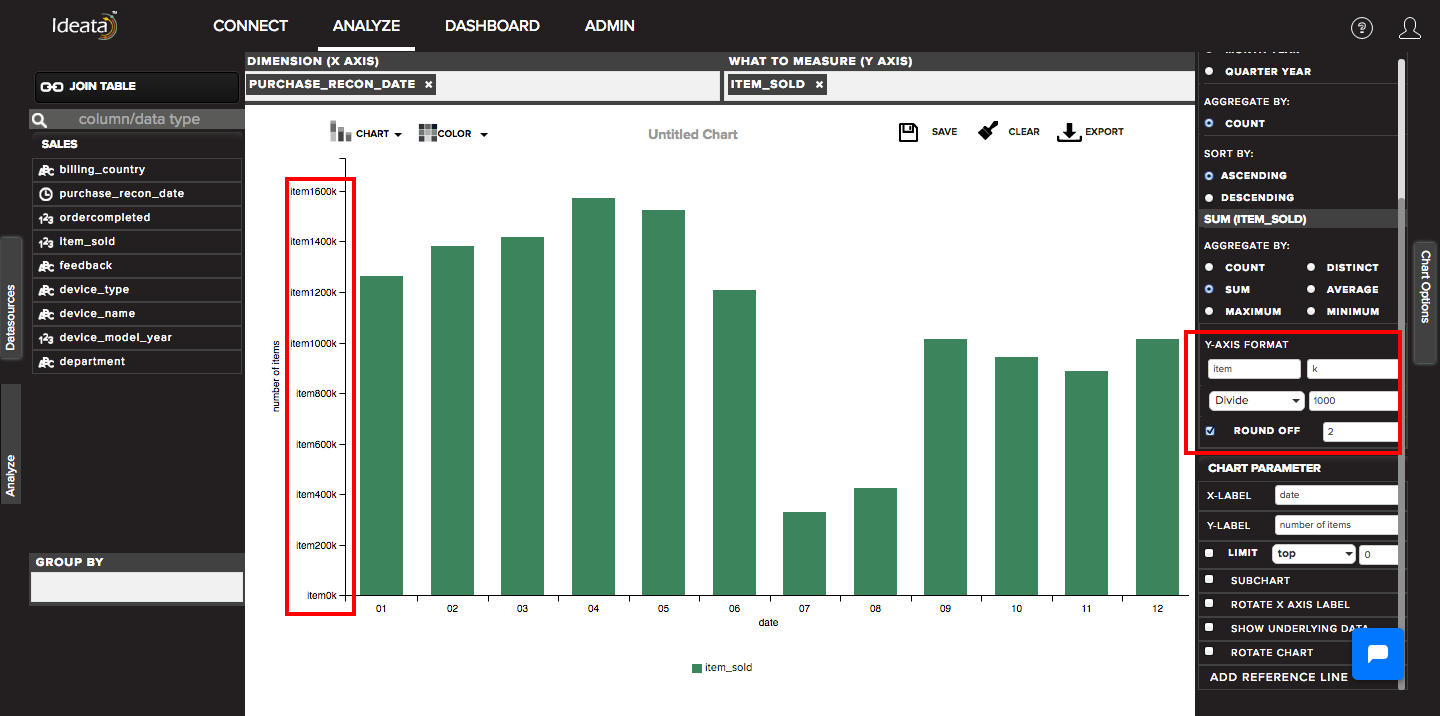# How to change y axis data format?

When we drag something in measures and if we want to change how the y axis datapoints looks in the chart, we can do it from y axis format options.

It provides various options such as:

• add prefix - we can add some text as prefix before y axis datapoints text. For example - add \$ before each datapoint such that 1000 appears as \$1000

• add suffix - we can also add some text or symbol as suffix after the dataspoints text. For example - add Rs. after each datapoint such that 1000 appears as 1000Rs.

• We can also apply some mathematical formula such as add, subtract, multiply or divide to change the numbers accordingly in y axis. for example if we get 50000 in y axis we can choose divide by 1000 and change it to 50

• round off - if there are decimal points in y axis we can also round them off by selecting round off check box and providing appropriate decimal palces in the text boxAll the changes done in the y axis format will automatically reflect on the y axis in chart area.